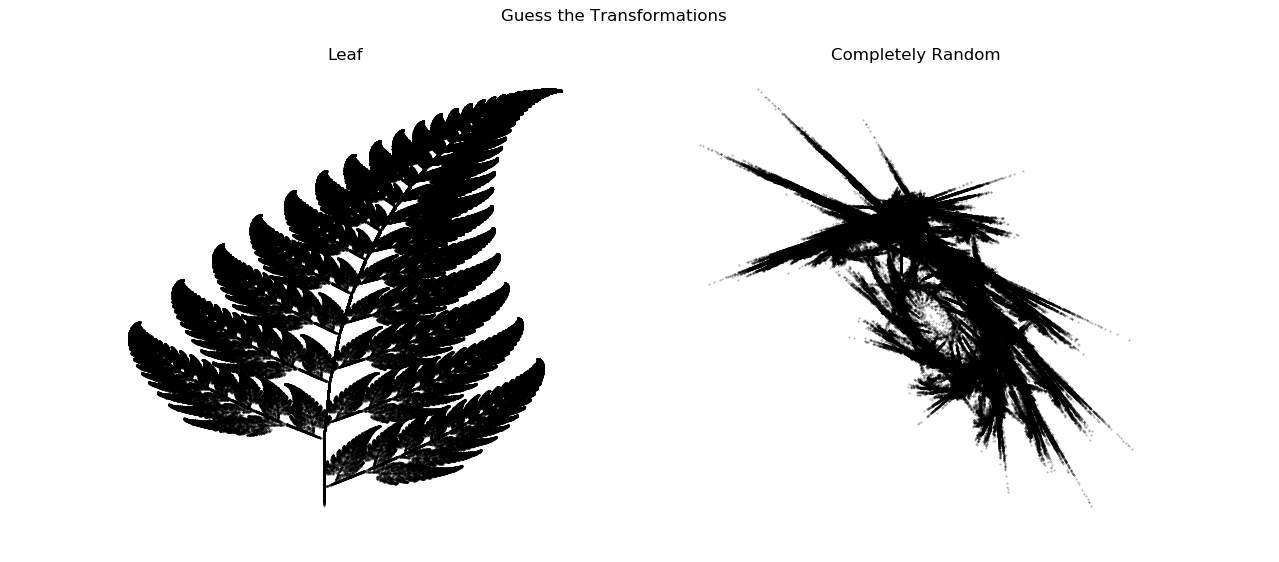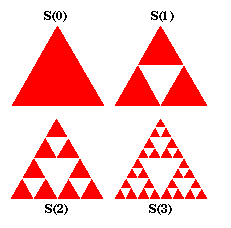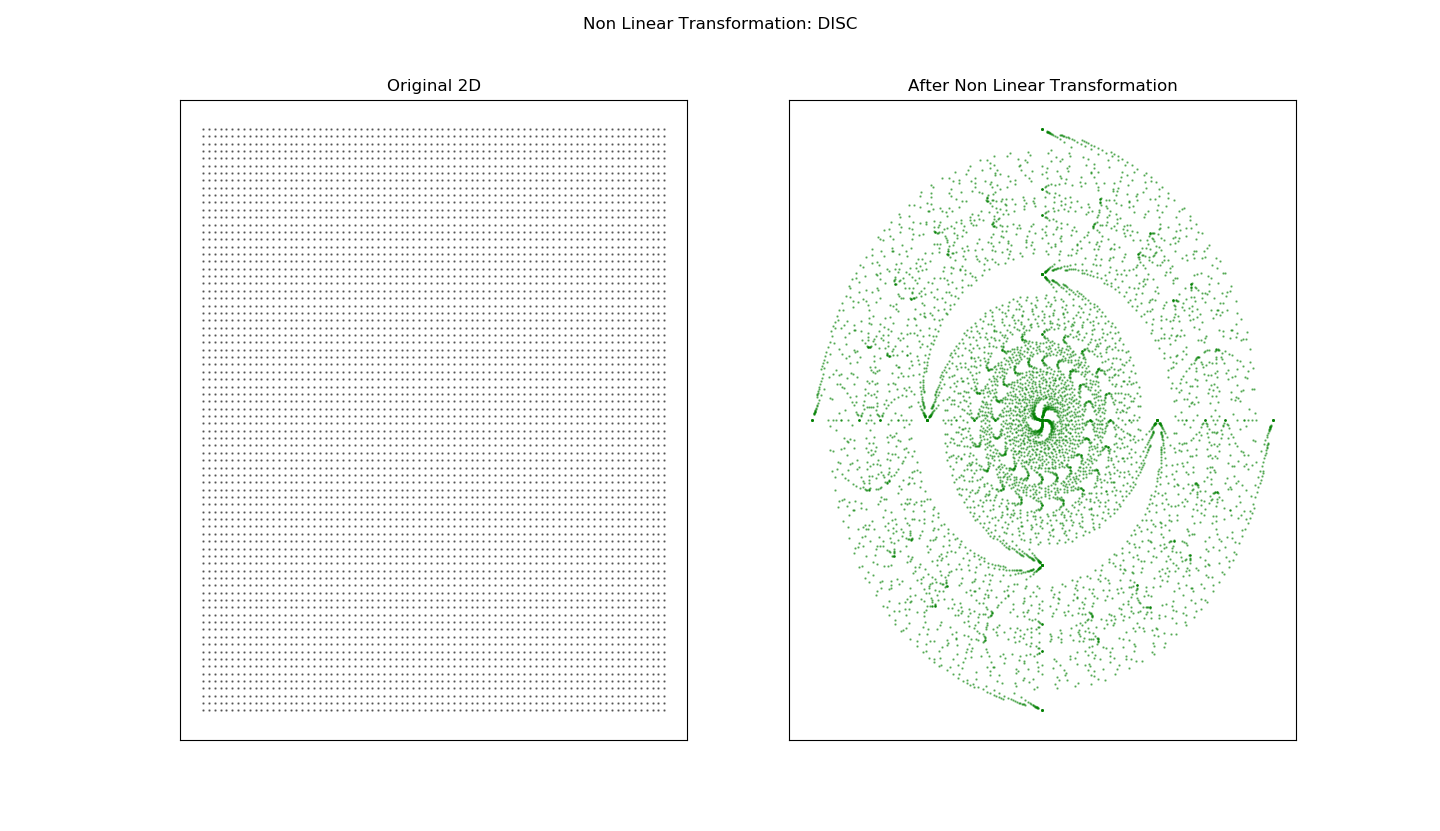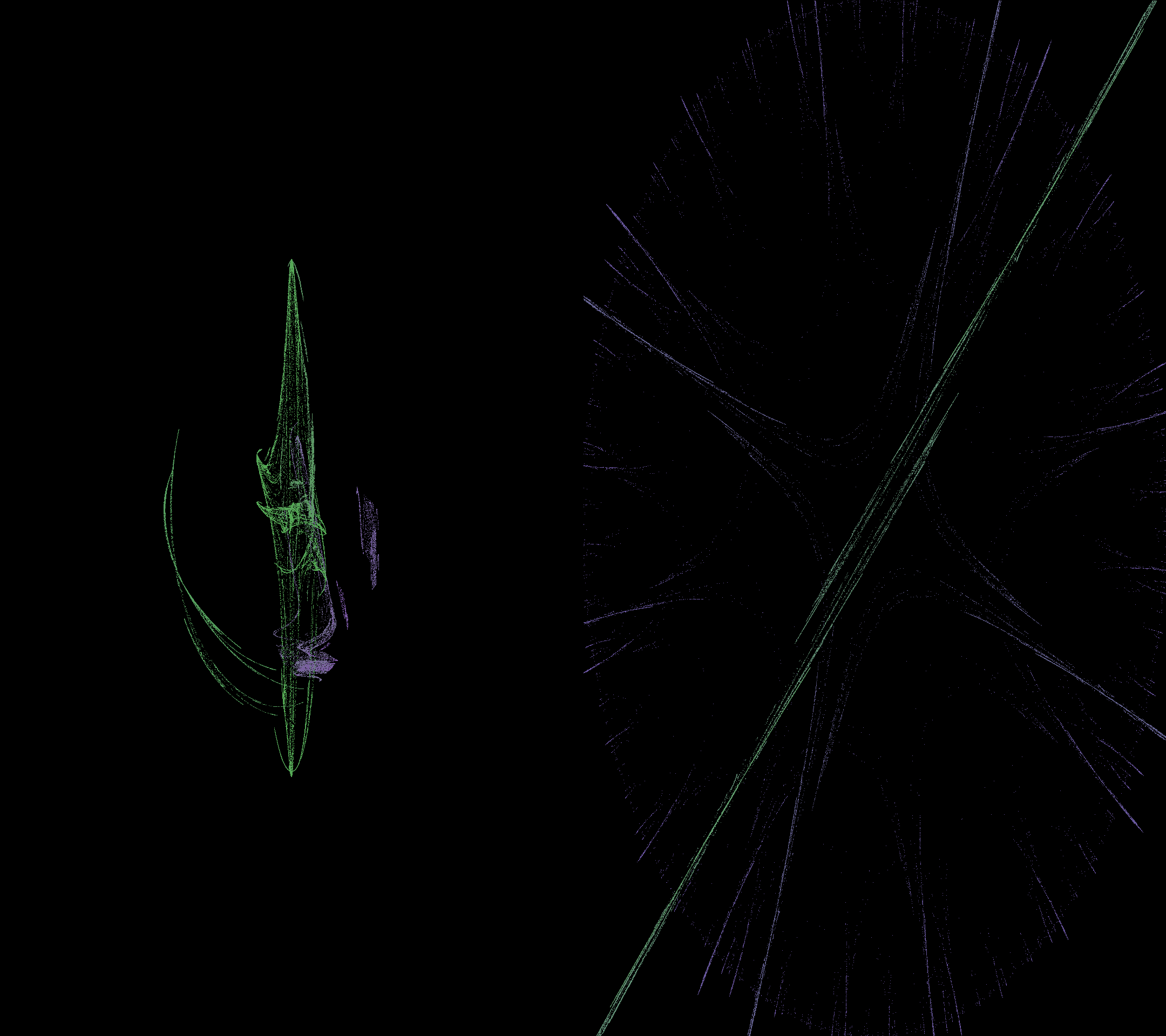# Fractal - It's an Art!

Continuing on the Fractals series, this article is devoted towards the artistic of Fractals. Fractals have been used extensively in the field of computer graphics and animation. Ofcourse, they have other highly useful applications as well in Finance, Communication etc. which we will cover in subsequent articles, but this one is my favourite. How a simple math application can generate such abstract art!

In my last article, I had touched upon the very important aspect of fractals, Iterativeness. Iterated Functions System (IFS) is better applied to design beautiful self-similar shapes and objects such as the ones shown below. One of them represents a leaf and other one is a shape that function chose itself through given probability distribution.One thing is common in the above shapes (and IFS in general), i.e, the Functions applied are linear in nature. The last article was concluded on the same note that what happens if we move beyond Linearlity?

I will structure this article by briefly recapping the IFS and then moving onto Non Linear Transformations. Subsequently, I will take a step further and design a highly generic IFS art which is a combination of Linearity and Non Linearity at the same time. So, sit back and enjoy the art of Fractals.

### Iterated Functions System (IFS) - A Recap

Earlier we talked about applying some sort of Linear functions iteratively. Too many mathematical words, huh! But what does that mean. Lets take a very simple example of Sierpinski Triangle (taken from my previous article)The Iterated Function System(IFS) for this image can be presented as:

1. Begin with a starting object
2. Reduce the object into half of the size and make 3 copies (3 function systems )
3. Align them in the shape of an equilateral triangle. (represented by the translation vector)
4. Go to step #2.
f_1(x) =
\begin{bmatrix}
.5 & 0 \\
0 & .5
\end{bmatrix}x
f_2(x) =
\begin{bmatrix}
.5 & 0 \\
0 & .5
\end{bmatrix}x + \begin{bmatrix}
.5 \\
0
\end{bmatrix}
f_3(x) =
\begin{bmatrix}
.5 & 0 \\
0 & .5
\end{bmatrix}x + \begin{bmatrix}
.25 \\
.433
\end{bmatrix}

A very simple Linear form of equations applied in tandem, iteratively, resulted in Sierpinski Triangle. So, let’s extend the concept to relatively complex structures and move away from linear world.

### Moving beyond Linearity

The idea of this series is not to delve too much into mathematics, but to enjoy the beauty of it. Here is a brief flavor of what happens when a Non-Linear Transformation is applied to two-dimensional coordinate system. In comparison, we will have a Linear Transformation as well, just to understand how different it looks.The images above can be described by 2 commonly used mathematical formations:

1. Sinusodial – If you look closely, the spacing between the data points are is not constant anymore. This spacing is defined by a Trigonometric function SINE
2. Disc – For a disc, the math is a little complex and it forms a more complicated non linear structure. But, it is aesthetically pleasant. Isn’t it?

### IFS on Non Linear Functions

So far, we had only discussed the application of 1 Non Linear function (eg: sine or disc). What if we apply several Non Linear functions using a probability, i.e. creating a system of Non linear equations to be applied iteratively. This form of art is generalized as Fractal Flame, and is highly used on computer graphics.These are 2 separate images, but due to full dark background, they seem to be inseparable. Nonetheless, quite elegant these images are. Aren’t they? Well, all of this is built using simple Python application. But with more professional tools, one can develop more beautiful arts.

For readers who are interested in detailed mathematics and how various non linear functions are used, please refer to the fantastic paper on Fractal Flame Algorithm.

### Fractals - The way forward

Ever heard of CGI in reference to movies? CGI stands for Computer Generated Imagery. Well, as you guessed it right by now, Fractals has a very strong hand in CGI. In motion pictures when you see random fire, or a galaxy or other animatioms, the idea of Fractals is everywhere.

Clearly, Benoit Mandelbrot opened up a whole new world of mathematics by introducing Fractals. It is not just in graphics and animation that we see its application. In the next articles, I will talk about its applications in various other fields where Fractals has gained momentum.